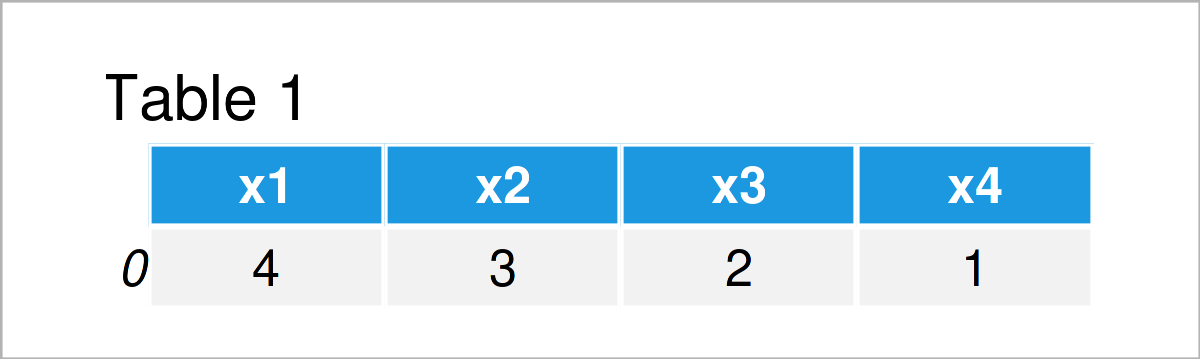# Convert pandas DataFrame to Dictionary in Python (Example)

In this tutorial you’ll learn how to create a dictionary from a pandas DataFrame in the Python programming language.

The article contains one example for the construction of a new dictionary from a pandas DataFrame. More precisely, the article consists of these content blocks:

You’re here for the answer, so let’s get straight to the example!

## Example Data & Software Libraries

We first need to load the pandas library, to be able to use the functions that are contained in the library:

`import pandas as pd # Import pandas`

Next, we’ll also have to create some exemplifying pandas DataFrame:

```data = pd.DataFrame({'x1': , # Create pandas DataFrame 'x2': , 'x3': , 'x4': }) print(data) # Print pandas DataFrame```Have a look at the previous table. It shows that our example data is constituted of one row and four columns.

## Example: Create Dictionary from pandas DataFrame Using to_dict() Function

The Python programming code below shows how to convert a pandas DataFrame to a dict object in the Python programming language.

For this task, we can use the to_dict function as shown in the following Python syntax:

```my_dict = data.to_dict() # Convert pandas DataFrame to dict print(my_dict) # Print dictionary # {'x1': {0: 4}, 'x2': {0: 3}, 'x3': {0: 2}, 'x4': {0: 1}}```

As you can see based on the previous Python console output, we have created a new dictionary called my_dict, which contains the values of our input data set.

## Video, Further Resources & Summary

Have a look at the following video on the Statistics Globe YouTube channel. I’m explaining the Python programming codes of this page in the video.

Furthermore, you may want to have a look at the other posts on this website. Please find a selection of tutorials about topics such as lists, data conversion, and indices below:

You have learned in this article how to transform a pandas DataFrame to a dictionary in the Python programming language. Tell me about it in the comments section below, in case you have further questions.

Subscribe to the Statistics Globe Newsletter